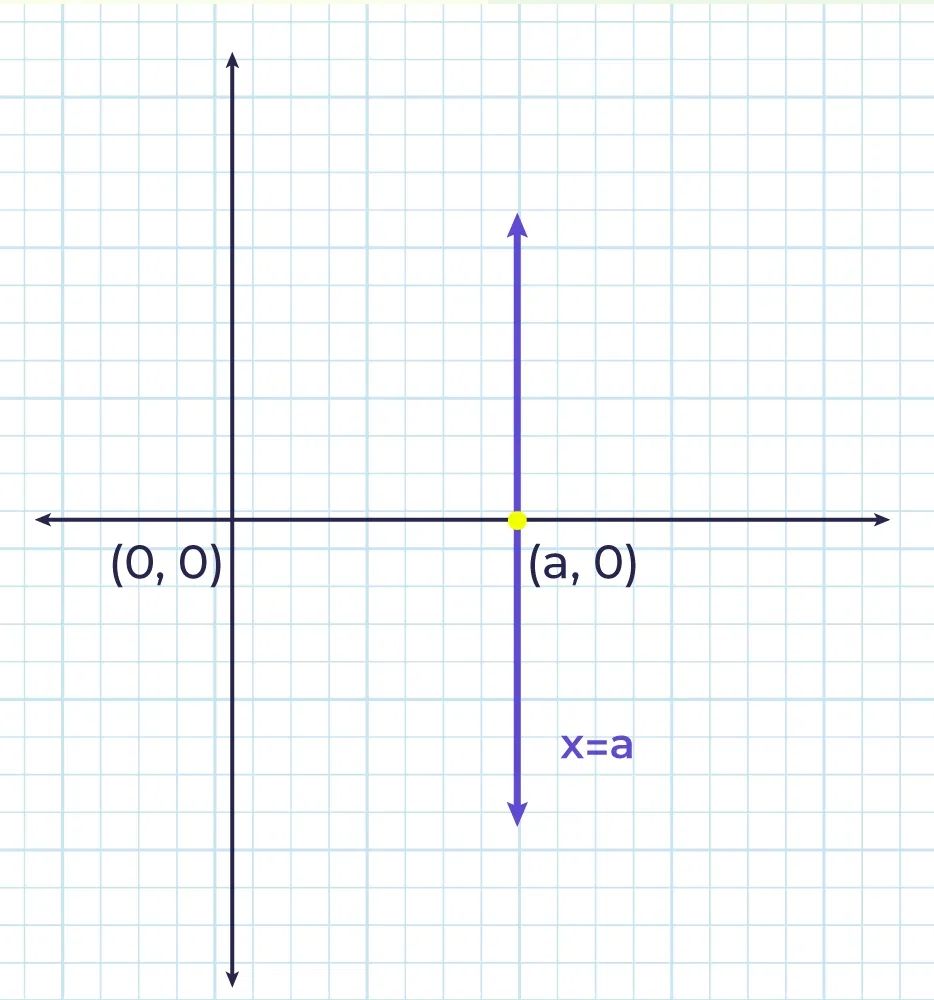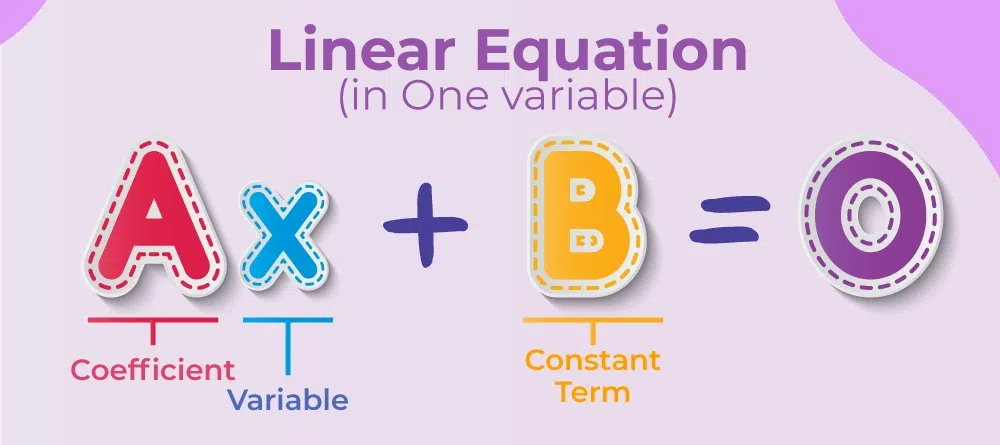Open In App
Related Articles
• CBSE Class 8th Maths Notes
• CBSE Class 8 Maths Formulas
• NCERT Solutions for Class 8 Maths
• RD Sharma Class 8 Solutions for Maths

# Linear Equations in One Variable

Linear equation in one variable is the equation that is used in algebra for finding unknown quantities. It is used for representing the conditions that are dependent on one variable. A linear equation in one variable is a linear equation i.e. the equation in which the degree of the equation is one, and it only has one variable. We can easily represent the linear equation in one variable in graphical form. We can use any variable such as x, y, z, etc to represent the linear equations. Other than the graphical method, the algebraical method is also used to solve linear equations in one variable. Linear equations are algebraic equations that are used to represent a straight line. It can also be considered as a polynomial of degree 1.

## What is Linear Equation in One Variable?

As we know a linear equation is an equation in which the degree of the polynomial is one, i.e. the highest power of the variable is one and a linear equation in one variable is a linear equation with only one variable. We can take any variable such as x, y, a, b, etc. Some examples of linear equations in one variable are,

• 2a + 3a = 20
• 5x + x = 12, etc.

The above equations are linear equations in one variable as they only have one variable and the highest degree of the variable is 1. These linear equations can be easily represented on the graphs and they represent the straight line which can be horizontal to the coordinate axis or vertical to the coordinate axis.We represent the mathematical condition using these equations as the condition “a number which is 5 less than twice of itself” is represented by the linear equation,

x = 2x – 5

where x is the unknown number.

In the above equation there is only one variable (x) and the degree of the variable is one thus, it is a linear equation in one variable.

## Standard Form of Linear Equation in One Variable

A linear equation in one variable can be expressed in the standard form,

ax+b = 0

where x is the variable and a and b are the constants involved. These constants (a and b) are non-zero real numbers. These equations have only one possible solution for the value of the variable.## Solving Linear Equations in One Variable

Linear Equations in One Variable can easily be solved by following the steps discussed below,

Step 1: Write the given equation in standard form and if a or b is a fraction then take the LCM of the fraction to make them integers.

Step 2: The constants are then taken to the right side of the equation.

Step 3: All the variables and the constant terms are then simplified to form one single variable term and one single constant.

Step 4: The coefficient of the variable is made 1 by dividing both sides with a suitable constant to get the final result.

Let’s understand the above steps with the help of the following example.

## Example of Solution of Linear Equation in One Variable

Solve the following linear equation in one variable.

Example: Solve 3x + 1/2 = (1/2)x – 13/2

Solution:

Step 1: Arranging in standard form and taking LCM

3x – (1/2)x + 1/2 + 13/2 = 0

(6x – x + 2 + 13)/2 = 0

6x – x + 2 + 13 = 0

Step 2: Transposing the constant to the right-hand side

6x – x = -2 – 13

Step 3: Simplification

5x = -15

Step 4: Make coefficient of x to 1

Dividing both sides by 5 we get,

5x/5 = -15/5

x = -3

This is the required solution.

## Linear Equations and Non-Linear Equations

As we have already learned that linear equations are the equations with the highest degree of “one” and they represent a straight line on the coordinate plane. Examples of linear equations are,

• 3x = 5
• x + y = 12
• 3x – 4y = 11, etc.

All these linear equations represent the straight line in coordinate planes.

All the equations which have a degree greater than one are called non-linear equations they represent curves in the coordinate plane. These equations have various applications in solving various problems of trigonometry, algebra, matrices, conic section, and others. Various types of non-linear equations represent various types of curves in the 2-D plane. Some of the common curves which we study are,

Circle: x2 + y2 = 49, this equation represents a circle in an x-y coordinate plane with a center at (0, 0) and a radius of 7 units.

Similarly,

All these equations discussed above are non-linear equations.

## Linear Equation in One Variable Example

Example 1: Solve for y, 8y – 4 = 0

Solution:

Solving for value of y,

Adding 4 to both sides of the equation ,

8y -4 + 4 = 4

8y = 4

Dividing both sides of equation by 8

y = 4/8

Simplifying the equation ,

y = 1/2

Example 2: Solve the equation in x, 3x +10 = 55

Solution:

Taking constants to RHS,

3x = 45

x = 15

Example 3: Solve the equation in x, 4/x5 -5 = 15

Solution:

4/x5 -5 = 15

⇒ 4x/5 = 15 + 5

⇒ 4x/5 = 20

⇒ x = 20×5/4

⇒ x = 25

Example 4: The age of Ravi is twice the age of his sister Kiran if the sum of their age is 24 find their individual age.

Solution:

Let the age of Kiran is x, then the age of Ravi is 2x

Given, the sum of their ages is 24

x + 2x = 24

⇒ 3x = 24

⇒ x = 8

Thus, the age of Kiran = x = 8 years

Age of Ravi = 2x = 2×8 = 16 years

Example 5: Akshay earns three times more than Abhay and if the difference in their salaries is Rs 5000 find their individual salaries.

Solution:

Let the salary of Abhay be x, then the salary of Akshay is 3x

Given, the difference in their salaries is Rs 5000

3x – x = 5000

⇒ 2x = 5000

⇒ x = 2500

Thus, the salary of Abhay = x = Rs 2500

The salary of Akshay = 3x = 3×2500 = Rs 7500

## FAQs on Linear Equation in One Variable

### Q1: What is a Linear Equation in One Variable?

Any equation with degree 1 and containing one variable is called a linear equation in one variable. They represent a straight line parallel to the coordinate axis.

### Q2: What is the Degree of Linear Equation in One Variable?

As we know Linear equations in One Variable are linear, i.e. the degree of the equation is ‘1’. We can say that in a linear equation, the highest power of the variable is 1.

### Q3: What are the Examples of Linear Equations in One Variable?

Various examples of linear equations in one variable are,

• 5a + 11 = 2a – 3
• 2x + x = 13 + 11
• y + 3y – 2y = 7 + 9

### Q4: Can a Linear Equation Have More than One Variable?

Yes, linear equations can have more than one variable, as all the equations with degree 1 are called linear equations. Thus, 2x+3y-7=0 and 3x+y-2z=8 are examples of linear equations that have more than one variable.

### Q5: How do you Solve Linear Equations in One Variable?

We can solve linear equations in one variable by simply following the following steps,

Step 1: Arrange the equation in standard form.

Step 2: Send all constants to the right-hand side.

Step 3: Simplify the equation to get the solution of the linear equation in one variable.

### Q6: What is the General Form of a Linear Equation in One Variable?

The general form of a linear equation in one variable is,

ax + b = 0

Where,

• x is the variable, and
• a, and b are constant and a can never be zero.